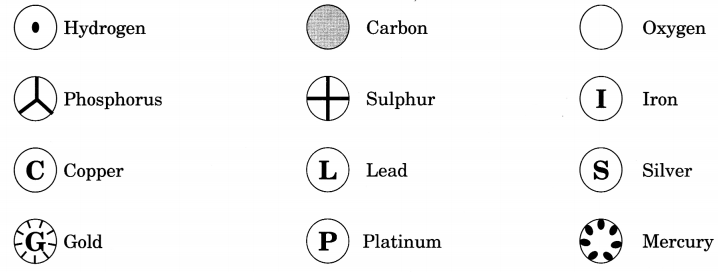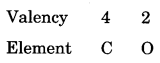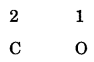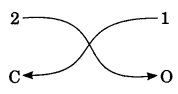On this page, you will find Atoms and Molecules Class 9 Notes Science Chapter 3 Pdf free download. CBSE NCERT Class 9 Science Notes Chapter 3 Atoms and Molecules will seemingly help them to revise the important concepts in less time.

## CBSE Class 9 Science Chapter 3 Notes Atoms and Molecules

### Atoms and Molecules Class 9 Notes Understanding the Lesson

1. The structure of matter has been a subject of speculation from a very early time. The ancient Indian and Greek philosophers regarded matter to be discontinuous and made up of infinitely small particles. Around the same period, Greek philosopher, Democritus, suggested that if we go on dividing matter into smaller parts, a stage would be reached when particles obtained cannot be divided further. He called these particles ‘Atoms’ meaning indivisible.

2. After Democritu’s death, little more was done with atomic theory until the end of the 18th century, when Antoine Lavoisier introduced modern chemistry to the world. He put forward two important laws of chemical combination which formed the basis of Dalton’s atomic theory which were published in 1808.

3. Laws of Chemical Combination: Whenever reactants react together to form the products or the elements combine together to form a compound, they do so according to certain laws. These laws are called “Laws of Chemical Combination”.

4. Laws of Conservation of Mass: Law of Conservation of Mass states that mass can neither be created nor destroyed in a chemical reaction.

5. Law of Constant Proportions: This law was stated by Proust as “In a chemical substance, the elements are always present in definite proportion by mass”. For example: In a compound such as water, the ratio of the mass of hydrogen to the mass of oxygen is always 1: 8, whatever the source of water. Thus, if 9 g of water is decomposed, 1 g of hydrogen and 8 g of oxygen are always obtained.

6. As the law of constant proportions is true, it helps us to calculate the percentage of any element in the given compound, using the following expression:

7. Dalton’s Atomic Theory : On the basis of laws of chemical combination, John Dalton, proposed that behaviour of matter could be explained using an atomic theory.

8. Postulates of Dalton’s Atomic Theory

• All matter is made of very tiny particles called atoms.
• Atoms are indivisible particles, which cannot be created or destroyed in a chemical reaction.
• Atoms of a given element are identical in mass and chemical properties.
• Atoms of different elements have different masses and chemical properties.
• Atoms combine in the ratio of small whole numbers to form compounds.
• The relative number and kinds of atoms are constant in a given compound.

9. Limitations of Dalton’s Atomic Theory

• It failed to explain how atoms of different elements differ from each other i.e., it did not tell anything about internal structure of the atom.
• It could not explain how and why atoms of different elements combine with each other to form compound- atoms or molecules.
• It failed to explain the nature of forces that hold together different atoms in a molecule.
• It did not make any distinction between ultimate particle of an element that takes part in reaction (atom) and ultimate particle that has independent existence (molecule).

Question 10.
Atom
An atom is defined as the smallest particle of an element which may not be capable of free existence. However, it is the smallest particle that takes part in a chemical reaction.

Question 11.
How big are atoms?
Atoms are very small, they are smaller than anything that we can imagine or compare with more than millions of atoms when stacked would make a layer barely as thick as a thin sheet of paper.

Atoms are very small in size. The size of an atom is expressed in terms of atomic radius. Atomic radius is measured in nanometers (nm).
$$1 \mathrm{nm}=\frac{1}{10^{9}} \mathrm{m}=10^{-9} \mathrm{m}$$

Radii of most of the atoms are the order of 0.1 nm or 10_1° m. For example, atomic radius of hydrogen is
0. 037 nm while that of gold atom is 0.144 nm.

Question 12.
Symbols used to represent atoms of different elements
Daltons suggested symbols for the atoms of different elements. He was the first scientist to use the symbols for elements in a quantitative sense. When he used symbol for an element he meant a definite quantity of that element, that is, one atom of the element.Question 13.
Modern Symbols of Elements
1. The symbol of an element is the “first letter and another letter” of the English or Latin name of the element. However, in all cases, the first letter is always capital and the other letter (if added) is always a small letter.
For example: Hydrogen is represented by ‘H’, oxygen by ‘O’, carbon by ‘C’ etc.

2. The necessity of adding another letter arises only in case of elements whose names start with the same
letter. However, the other letter added is not always the second letter of the name. Further, the other – letter added may be a letter from the Latin name of the element.

For example: Carbon, Calcium, Cobalt, Chlorine and Copper all start with the first letter ‘C’ Hence carbon is represented by C and calcium is represented by Ca, cobalt by Co, chlorine by Cl and copper by Cu

 Element Symbol Element Symbol Element Symbol Aluminium A1 Copper Cu Nitrogen N Argon Ar Fluorine F Oxygen O Barium Ba Gold Au Potassium K Boron B Hydrogen H Silicon Si Bromine Br Iodine I Silver Ag Calcium Ca Iron Fe Sodium Na Carbon C Lead Pb Sulphur S Chlorine Cl Magnesium Mg Uranium U Cobalt CO Neon Ne Zinc Zn

Question 14.
Atomic Mass
The atomic mass of an element is the relative mass of its atoms as compared with the mass of an atom of carbon -12 isotope taken as 12 units.
$$\text { Atomic mass }=\frac{\text { Mass of } 1 \text { atom of the element }}{\frac{1}{12} \text { of the mass of an atom of Carbon-12 }}$$

Since atomic masses are relative masses, they are pure numbers and are often given without units. In case of most of the elements, all the atoms of the elements do not have same relative mass. The atoms of an element having different relative masses are called isotopes. For example: Chlorine contains two types of atoms having relative masses 35 u and 37 u and their relative abundance is 3:1.
$$\text { Atomic mass of chlorine }=\frac{35 \times 3+37 \times 1}{4}=35.5 \mathrm{u}$$

Question 15.
What is a Molecule?
A molecule can be defined as the smallest particle of an element or a compound that is capable of an independent existence and shows all the properties of that substance.

Question 16.
Molecule of an element
Molecule of an element means one, two or more atoms of the same element existing as one species in the free state.
(i) Monoatomic molecules: Noble gases like helium, neon, etc., exist as single atom. Hence, their molecules are monoatomic.

(ii) Diatomic molecules: For example, in a molecule of hydrogen, two atoms of hydrogen exist together. Its molecule is, therefore, represented by H2, i.e., it is a diatomic molecule.

(iii) Triatomic molecules: For example, in a molecule of ozone, three atoms of oxygen exist together as one species. Hence, it is triatomic with formula O2

(iv) Tetratomic molecules: For example; Phosphorus P4 is a triatomic molecule.

Molecules containing more than four atoms are generally called polyatomic.

The number of atoms present in one molecule of the substance is called its atomicity.

Molecules of a compound: Atoms of different elements join together in definite proportion to form molecules of compounds. For example, H2O represents the molecule of a compound in which two atoms of hydrogen are combined with one atom of oxygen, or hydrogen and oxygen are combined in the fixed proportion, i.e., 1 : 8 by mass.

Molecular mass: The molecular mass of a substance (an element or a compound) may be defined as the average relative mass of a molecule of the substance as compared with mass of an atom of carbon (C-12 isotope) taken as 12 u.
$$\text { Molecular mass }=\frac{\text { Mass of } 1 \text { molecule of the substance }}{\frac{1}{12} \text { of the mass of an atom of } \mathrm{C}-12}$$

For example, molecular mass of CO2 is 12 x 1+ 16 x 2 = 44.
Formula mass: Formula mass of an ionic compound is obtained by adding atomic masses of all the atoms in a formula unit of the compound.
For example, formula mass of potassium chloride (KCl)
= Atomic mass of potassium + atomic mass of chlorine
= 39 + 35.5
= 74.5

Question 17.
What is an ion?
An atom or a group of atoms which contains positive or negative charge are called ions.

• A positively charged ion called ‘cation’.
• A negatively charged ion is called ‘anion’.
• The ions consisting of only single atoms are called monoatomic ions.
• The ions consisting of a group of atoms is called polyatomic ion.

Question 18.
Writing chemical formula
Chemical formula of a compound represents the actual number of atoms of different elements present in one molecule of the compound. For example, chemical formula of water is H2O.

Valency is defined as the combining capacity of the element. It is equal to the number of hydrogen atoms or number of chlorine atoms or double the number of oxygen atoms with which one atom of the element combines.

For example, valency of oxygen is 2. This means that one atom of oxygen can combine with two atoms of hydrogen. Hence, the formula of the compound formed is H2O.

Rules for writing the chemical formula

• Formula of compound is given by writing the symbols of constituent elements side by side.
• Symbol of the more metallic element is written first in the formula.
• Number of atoms of each of the constituent elements present in the molecule is indicated by subscript.
• When either of the ions or both the ions are polyatomic and their valency is more than 1, we enclose the polyatomic ions in brackets. No brackets are riecessary if the valencies of polyatomic ions are 1.
• While writing formula of a compound if the valency numbers have a highest common factor [H.C.F.], divide the valency numbers by H.C.F. to get a simple ratio between the combining elements.

The simplest compounds, which are made up of two different elements are called binary compounds and formula can be written by criss crossing the valencies of elements present in a molecule of the compound. Formula of the compound can be derived by following steps

Step – 1: Write the constituent elements and their valencies as shown.Step – 2: Reduce the valency numerals to the simplest whole numbers by dividing by some common factor, if anyStep – 3: Criss-cross the reduced valency numerals and write them as subscripts at the bottom right-hand side of the symbols.The subscript 1 is not written. Thus, the formula of the compound is CO2.

Mole concept: A mole of particles (atoms, ions or molecules) is defined as that amount of the substance which contains the same number of particles as there are C-12 atoms in 12 g, i.e., 0.012 kg of C-12 isotope.

Te number of particles (atoms, molecules or ions) present in 1 mole of any substance is fixed, with a value 6.022 x 1023. This number is called avogadro’s number. Avogadro’s number (NA or N0) = 6.022 x 1023

The volume occupied by one mole of molecules of a gaseous substance is called molar volume or gram molecular volume.### Class 9 Science Chapter 3 Notes Important Terms

Element: A chemical substance that cannot be decomposed by chemical means into simpler parts. It contains same kind of atoms. There are 118 elements known, out of which 94 are natural.

Compound: When two or more atoms of different elements chemically combine, the molecule of a compound is obtained. The examples of some compounds are water, ammonia, sugar, etc.

Molar mass: Molar mass is the sum of the atomic masses of the elements present in a molecule. It is obtained by multiplying the atomic mass of each element by the number of its atoms and adding them together.

Ion: Any atom or molecule which has a resultant electric charge due to loss or gain of valence electrons.

Valency: It is the capacity of atoms of a given element that tend to combine with, or replace atoms of hydrogen. In HCl gas, valency of chlorine is 1.

Atomicity: It corresponds to the number of atoms present in a given molecule of an element. For example, ozone (O3) has an atomicity of 3 and benzene (C6H6) has an atomicity of 12.

Mole: [Historically the word ‘mole’ was introduced about hundred years back by Wilhelm Ostwald. He derived this word from the Latin word ‘moles’ meaning ‘ heap or a pile’]. A mole is the amount of a substance that contains as many elementary entities (atoms, molecules or other particles) as there are atoms in exactly 0.012 kg of the carbon-12 isotope.